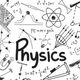• 1514

# Nicolaus Copernicus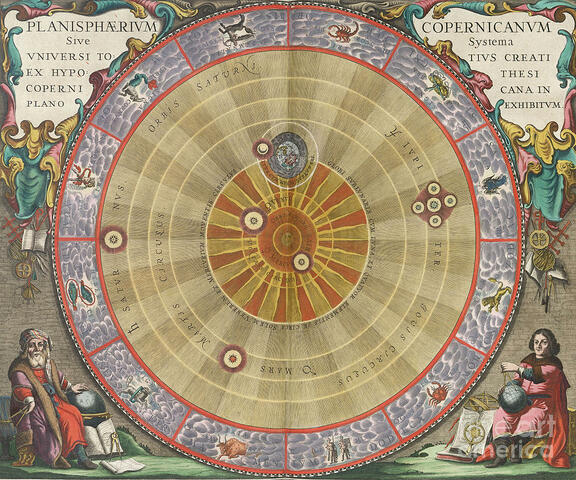Nicolaus Copernicus wrote the treatise commonly referred to as “Commentariolus”. In which he postulates that the planets, including the earth, orbit around the sun. Copernicus was unsure of this theory and his findings were not published until 1543, a year after his death.
Up until this point it was comonnly believed that the earth was the certre of the universe and all the celestial bodies, including the sun, orbitted around it.
• # Willebrord SnellSnell rediscovers the laws of refraction and creates an equation, known as Snell's Law (n1/n2 = sin α2/sin α1), that can be used to predict how the path of light changes as it moves between mediums of differing densities.
• # Galileo Galilei cont (text limit)

He also established the principle of inertia. Stating that a body in motion would travel in a straight line and at a constant speed unless acted upon by an external force.
While working with pendulums he discovered that the length of a pendulum is equal to its period of oscillation squared regardless of its mass.
These early concepts of measurable rules by which the universe operates would go on to become essential building blocks for later scientists.
• # Galileo GalileiGalileo established the law of falling bodies. Through experimentation, he discovered that gravity accelerates objects at the same speed despite their differing masses.
He also discovered that gravity accelerates at a constant rate, where distance equals time squared. This consistent gravitational acceleration is what causes projectiles to travel in a curved (parabola) trajectory.
He was placed under house arrest by the church and died eight years later in 1642.
• # Robbert Hooke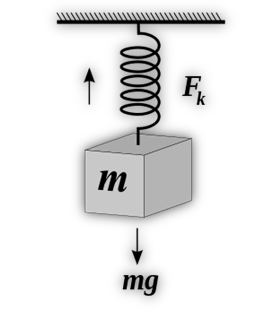In 1660, Robert Hooke discovered the theory of elasticity that would come to be known as Hook’s Law (F=kx). It states that the tension force of a spring will increase in proportion to the distance it is stretched.
This discovery allows for the measurement of energy (force) stored within a deformed material.
• # Robbert Hooke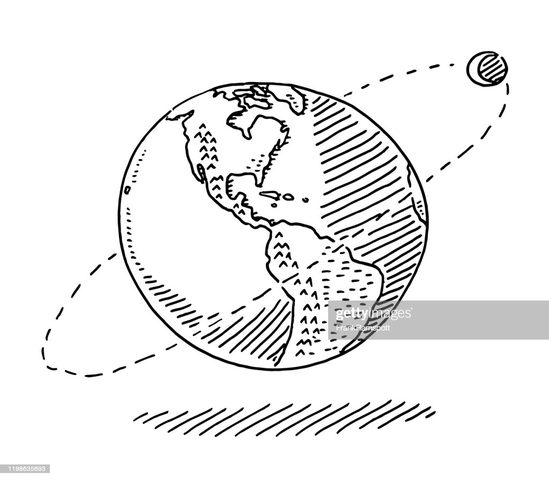Robert Hooke gives a lecture on the properties of gravity. He states that all celestial bodies have their own gravity, they would all just travel in straight line if there were no gravitational pull between them, and the gravitational pull between two objects increases the closer they are.
Another important step in the slowly growing understanding of gravity's effect on our surrounding universe.
• # Issac NewtonIssac Newton Presents his law of universal gravitation (F = G(m1m2)/r^2). He also creates his three laws of motion. Both the law of universal gravitation and the laws of motion build upon the previous works of other (Hooke and Galileo) but flesh out the ideas and turns them into measurable quantities.
• # Isaac Newton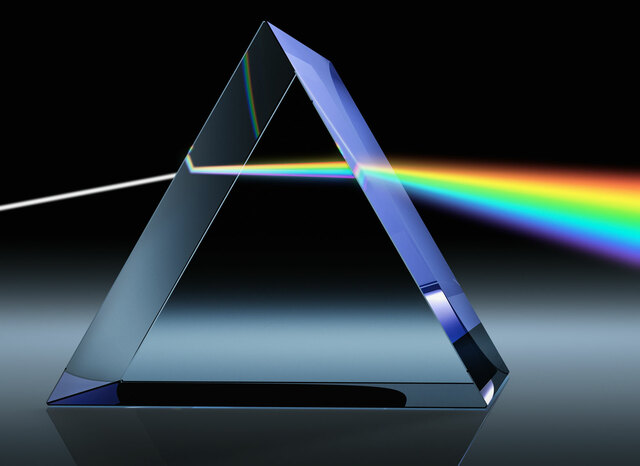Newton publishes "Opticks" in which he explains his various experimentations on light.
By passing light through a prism he shows that white light is actually made of a spectrum of colours. This spectrum of colour can even be reformed back into white light be being refracted back through a second prism. Showing that light consists of more than just a solid beam and explaining where our observable colours comes from. He also believed that light was made out of corpuscles (particles).
• # Charles-Augustin de Coulomb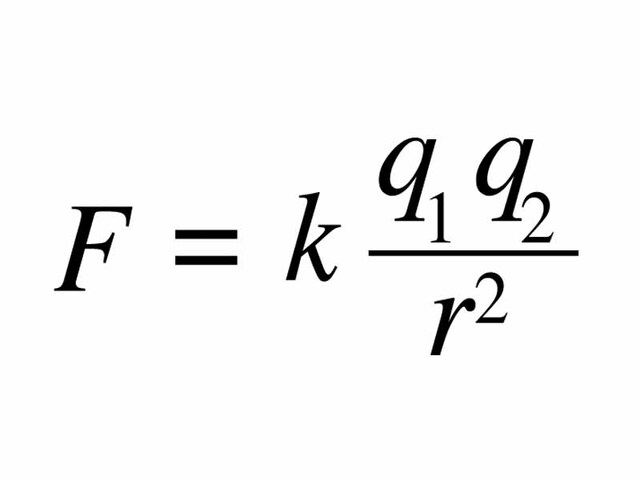Coulomb establishes Coulomb’s Law (F=k(q1q2)/r^2), which predicts the electrostatic force of attraction or repulsion between two charges. This allows us to measure the forces in play (attraction or repulsion) between particles and magnets.
• # Alessandro Volta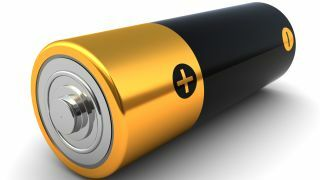Using alternation discs of zinc and copper, separated by a divider (cloth or card) moistened in brine, Volta created the first reliable battery. This led to a boom in electrical experimentation as now there was finally a reliable source of electricity. Volta also developed a means of measuring both electric potential and electric charge. This was known as Volta's Law of Capacitance.
• # Thomas YoungThomas young performs the double slit experiment. It produced and interference pattern that proved that light does indeed travel as a wave.
This was thought to disprove Newton's theory of light being a particle but they were both right. Wave-Particle duality would not be discovered until much later.
• # Andre-Marie Ampere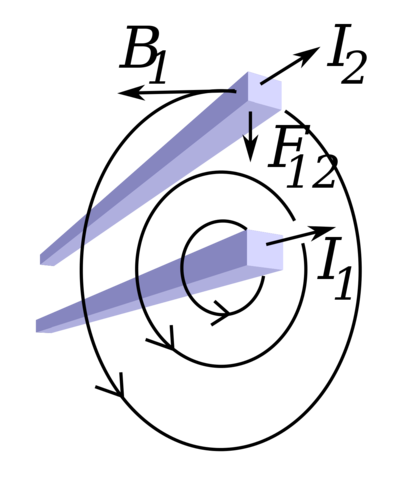While running electricity through two parallel wires, Ampere discovered that the wires acted like magnets. Attracting and repelling each other depending on what way the current traveled. He created Ampere’s circuital law to measure the strength of this new magnetic field. Using this equation (∫B.dl = μ0I) we can measure the strength of the electromagnetic field produced by an electric current.
• # Andre-Marie AmpereAndre-Marie Ampere published the “The mathematical theory of electrodynamic phenomena deduced solely from experiment”. In which he proposed the existence of an “electrodynamic particle”. A particle that would later be named the electron.
• # Georg OhmOhm publishes "Die Galvanische Kette, Mathematisch Bearbeitet". In which he describes the equation that would come to be known as Ohm's Law (I=V/R). A very important equation when dealing with the physics of electricity as it assists the user in finding the current (I), voltage (V), or resistance (R) in a circuit.
• # James Joule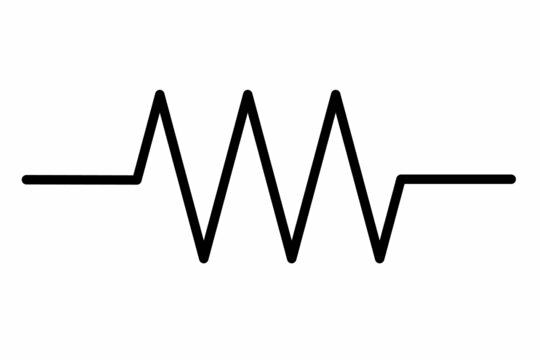James Joule invents “Joule’s Law” (P = I^2R) which states that the heat produced by an electric circuit is equal to the circuit’s current squared multiplied by the resistance. He published his findings in his 1845 paper “On the mechanical equivalent of heat”. The same paper also put forward the idea that both heat and mechanical work are forms of energy. This shows the transition from one form of energy to another.
• # Alexander Kirchhoff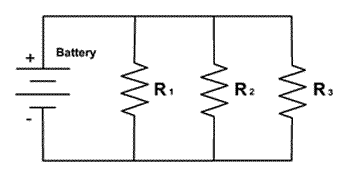Kirchhoff established what would come to be called Kirchhoff’s Circuit Laws.
First Law: The current flowing into a node or junction must be equal to the current flowing out of it.
Second Law: The voltage supplied to a circuit must equal the voltage used by the components in that circuit.
This gives us a better understanding of how circuits work, especially parallel circuits.
• # Albert Michelson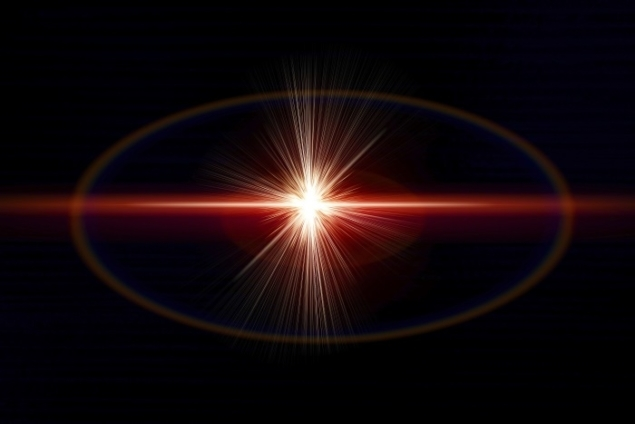Using a rotating mirror mechanism, Michelson sought to measure the speed of light. The final result of his experiment was a measurement of 299,944±51 km/s (while in a vacuum). This would remain the most accurate measurement of the speed of light for quite some time. Michelson would take part in another experiment in 1931 to get a more accurate measurement but died before its completion.
• # Thomas Edison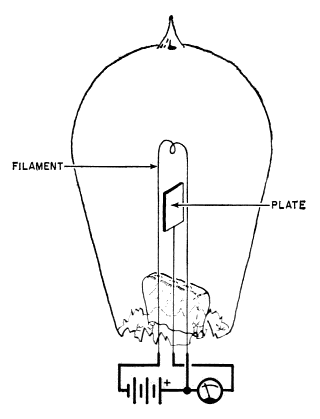Thomas Edison discovered that while in a vacuum electrons would flow from a heated element to a cooler piece of metal but only in one direction. This was termed the Edison Effect but is today known as Thermionic Emission. This shows that, given enough energy (heat), an electron can break free of its usual bonds.
• # Edward MorleyEdward Morley worked with Albert Michelson in an attempt to measure the movement of luminiferous aether. A substance believed to be the medium through which light traveled through space as a current. They were unable to measure the aether and ended up disproving its existence all together.
The Michelson-Morley experiment helped show that while light does act like a wave it does not require a medium through which to traverse space.
• # Nikola Tesla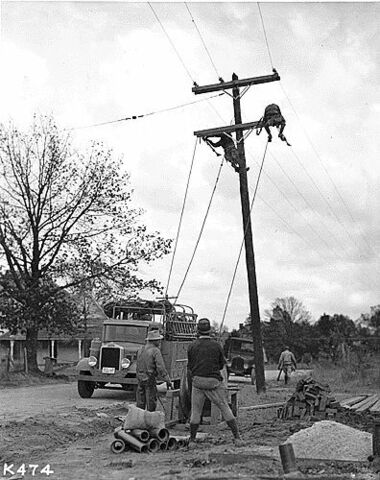Nikola Tesla invents a new electricity distribution system, alternating current (AC). Alternating current had far less power loss while being transmitted over long distances than the currently popular direct current (DC).
• # Marie Currie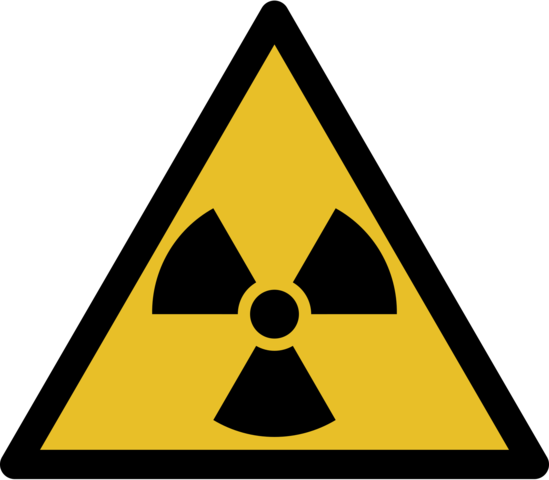Marie Currie wins a Nobel Prize in physics for her work on radiation. Her studies had shown that the amount of radiation emitted from uranium was determined by the amount of uranium and not it’s composition. This showed that the radiation came from the uranium's atom and not from a chemical reaction. She shared this award with Pierre Currie and Henri Becquerel.
• # Niels BohrNiels Bohr proposed a model of the atom in which the electrons orbit around the nucleus but only at set orbits. Electrons are able to move up and down these orbits as they gain or lose energy but they can not exist in the areas between the orbits. Disappearing from one orbit and reappearing in another via a quantum leap.
This explained why the negatively charged electrons did not get drawn into the positively charged nucleus.
• # Max PlanckMax Planck wins a Nobel Prize for the creation of Quantum Physics.
While doing experiments on thermodynamics, Planck found that the light emitted by heated objects is made of miniature packets (quanta) of energy. The amount of energy they carry being determined by their frequency.
This completely changed physics. A field that many thought was almost completely exhausted of any new possible discoveries.
• # Werner Heisenberg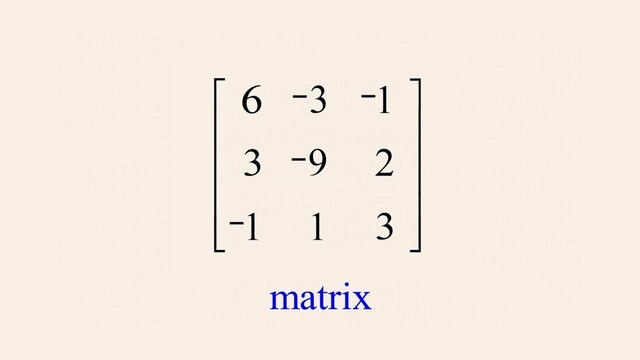Heisenberg discovered a way to use matrices with quantum mechanics. This was considered a much easier method of dealing with complicated equations.
• # Werner HeisenbergWerner Heisenberg publishes one of his most famous works, his “uncertainty principle”. It states that you can not know both the exact velocity and the exact position of an object at the same time.
• # Edwin Hubble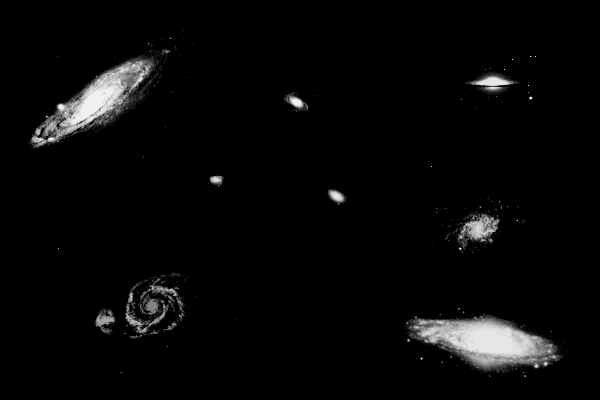Sound waves change due to the movement of their source. This is called the Doppler effect. Light waves act similarly if their source is moving as well. As a star moves away from us, its light shifts towards the red end of the spectrum.
Hubble put this red shift data into a chart and came up with the Hubble’s Law equation (v=Hd). Hubble’s Law is used to measure the speed at which a star is moving away from the earth.
This discovery led rise to the theory of the expanding universe.
• # Aage Bohr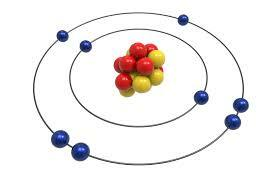Aage Bohr publishes “The Rational State of Atomic Nuclei”, in which he predicted the current model of the atomic nucleus. One which consists of protons and neutrons in constant motion.